# Dividing Fractions Worksheet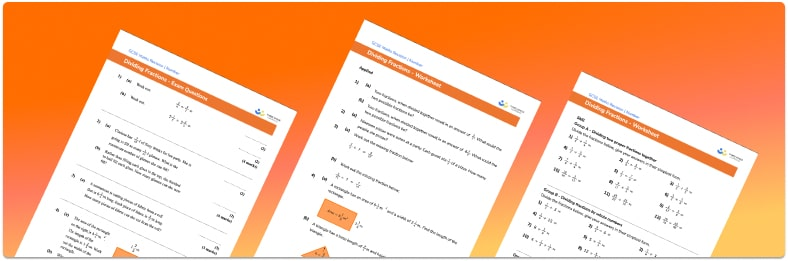Help your students prepare for their Maths GCSE with this free dividing fractions worksheet of 44 questions and answers

• Section 1 of the dividing fractions worksheet contains 36 skills-based dividing fractions questions, in 3 groups to support differentiation
• Section 2 contains 4 applied dividing fractions questions with a mix of fractions word problems and deeper problem solving questions
• Section 3 contains 4 foundation and higher level GCSE exam style dividing fractions questions
• An answer key and a mark scheme for all dividing fractions questions are provided
• Questions follow variation theory with plenty of opportunities for students to work independently at their own level
• All questions created by fully qualified expert secondary maths teachers
• Suitable for GCSE maths revision for AQA, OCR and Edexcel exam boards
• To receive this resource and regular emails with more free resources, blog posts and other Third Space updates, enter your email address and click below.

• This field is for validation purposes and should be left unchanged.

You can unsubscribe at any time (each email we send will contain an easy way to unsubscribe). To find out more about how we use your data, see our privacy policy.

### Dividing fractions a glance

Division of fractions is more straightforward than adding or subtracting fractions, because a common denominator is not required. It is important however that students are confident with equivalent fractions and are able to simplify fractions. Fraction multiplication is a prerequisite to dividing fractions.

Students need to be able to divide integers by fractions, fractions by integers, and divide a fraction by another fraction. Dividing an integer by a fraction is the most intuitive place to start, as we can look at how many times the fraction fits into the whole amount (e.g. how many halves are in 3?). This develops the idea of using the reciprocal (dividing by a half is the same as multiplying by 2).

To divide a pair of fractions, we invert the second fraction (essentially, flip the numerator and denominator) to find the reciprocal. We then multiply the first fraction by the reciprocal of the second fraction and cancel common factors in order to write the fraction in its lowest terms.

Fraction division can be extended to mixed numbers (sometimes called mixed fractions). A mixed number has both an integer (whole number) part and a proper fraction part. We can convert mixed numbers into improper fractions in order to perform calculations with them.

Looking forward, students can then progress to additional number worksheets, for example a or aFor more teaching and learning support on Number our GCSE maths lessons provide step by step support for all GCSE maths concepts.

## Related worksheets

Converting Mixed Numbers To Improper Fractions Worksheet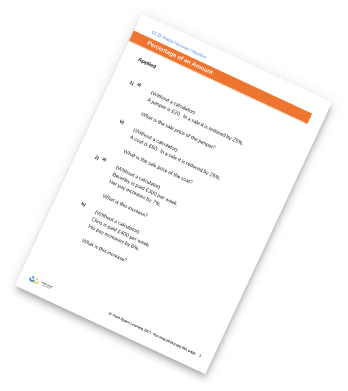Adding And Subtracting Fractions Worksheet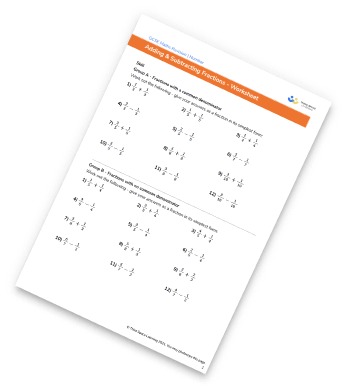Multiplying And Dividing Fractions Worksheets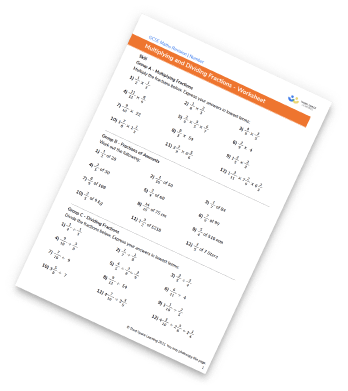Fractions Of Amounts Worksheet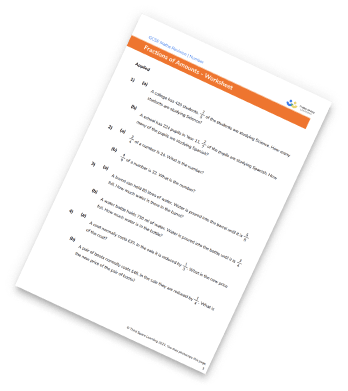## Do you have KS4 students who need more focused attention to succeed at GCSE?There will be students in your class who require individual attention to help them succeed in their maths GCSEs. In a class of 30, it’s not always easy to provide.

Help your students feel confident with exam-style questions and the strategies they’ll need to answer them correctly with our dedicated GCSE maths revision programme.

Lessons are selected to provide support where each student needs it most, and specially-trained GCSE maths tutors adapt the pitch and pace of each lesson. This ensures a personalised revision programme that raises grades and boosts confidence.

Find out more